# Annex X.8 - LOCAL AND GLOBAL VECTORIAL QUANTITIES

This annex shows as a remember the most frequent relations from the theory of vector fields which are used throughout the entire paper, with the specification that the notion’s denominations are the ones from the mathematics field, some of them being redefined in the present paper.

1. The gradient of a scalar field (x,y,z) is a vector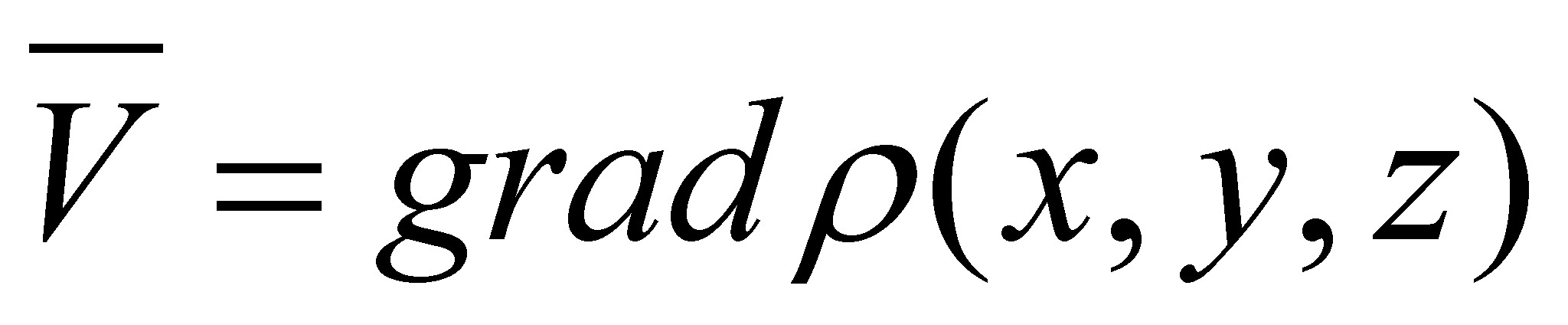given by the following relation: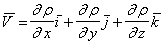(X.8.1)

where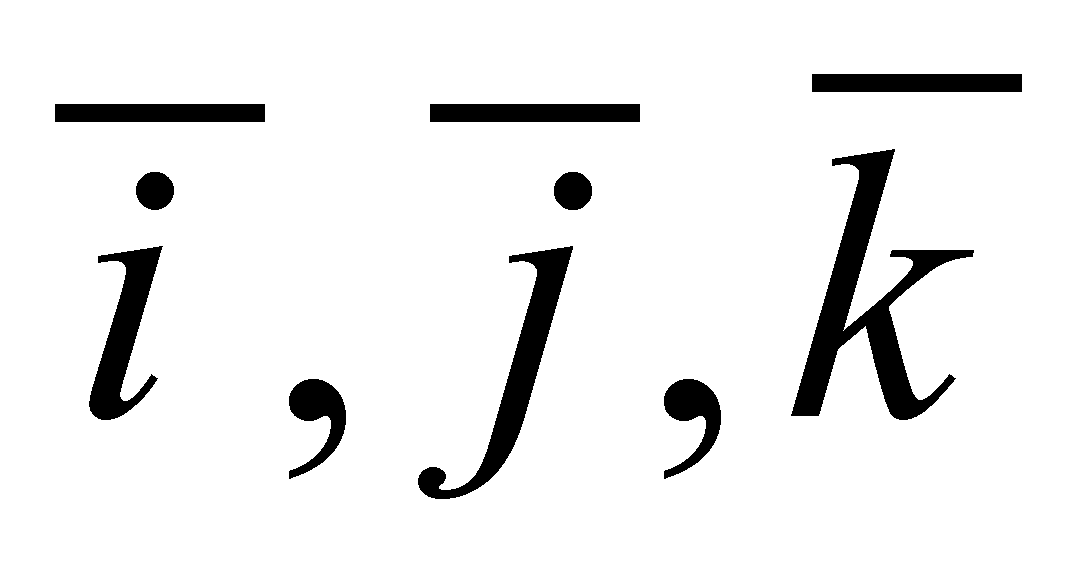are the versors of the axes X,Y,Z.

1. The elementary flux of the vector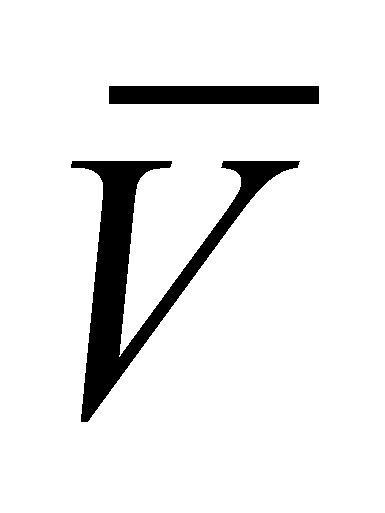is named the product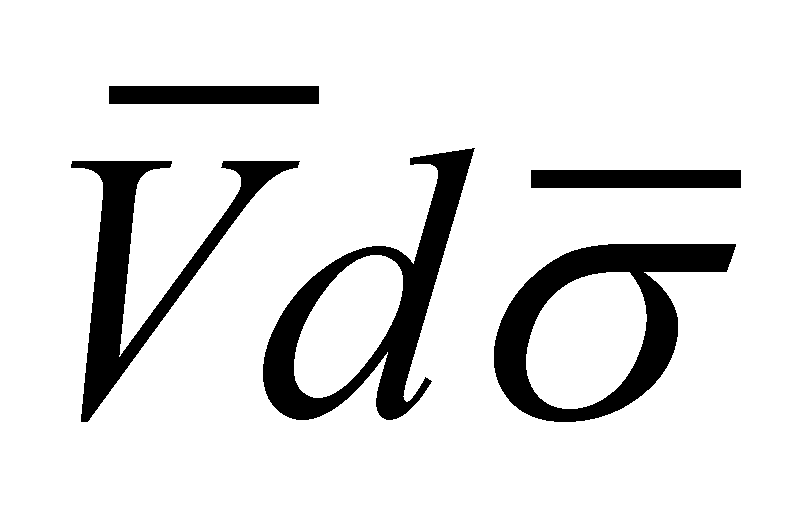, where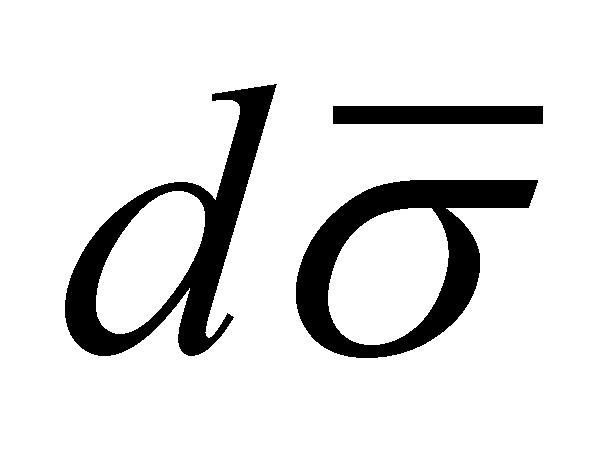is the oriented area element (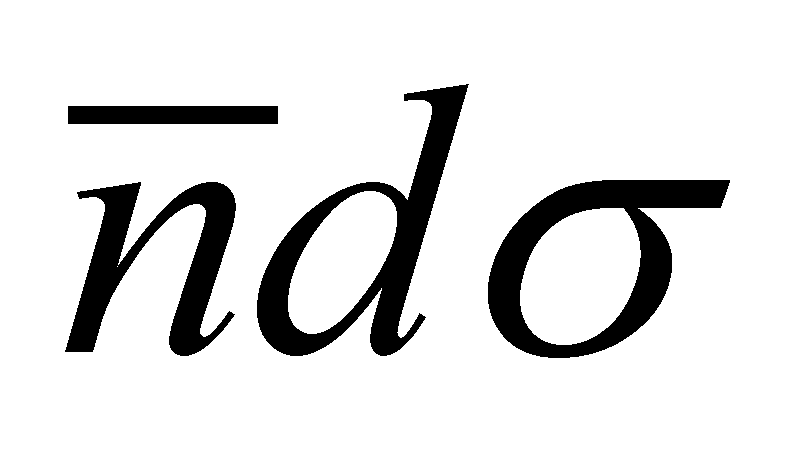). If the area element surrounds a point P(x, y, z), then, the elementary flux will be in point P.

1. Total (global) flux of the vectorthrough any surface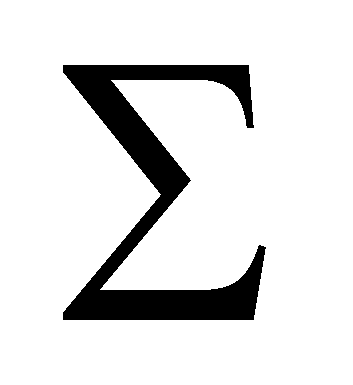is: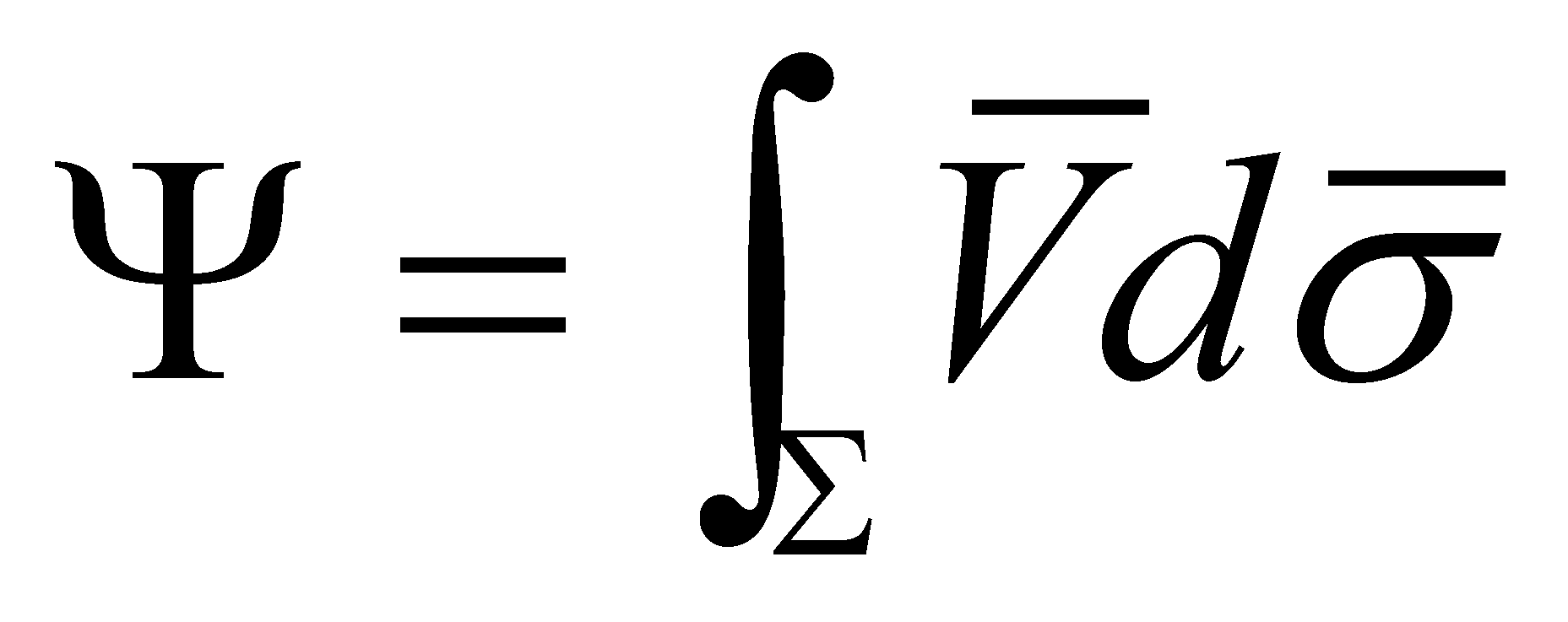(X.8.2)

1. The total flux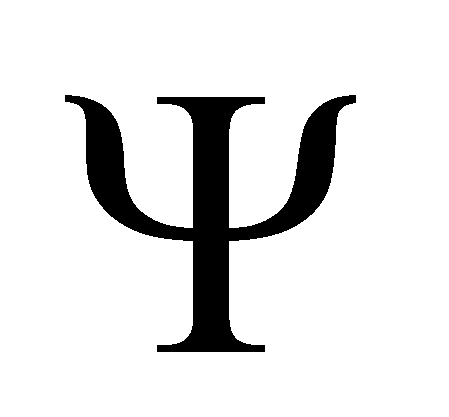through a confined surfacewhich is the boundary of a volume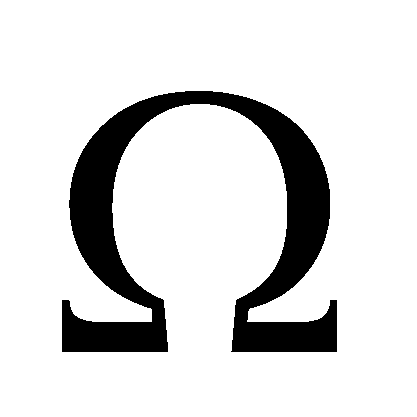is also named the productivity of the volume. The ratio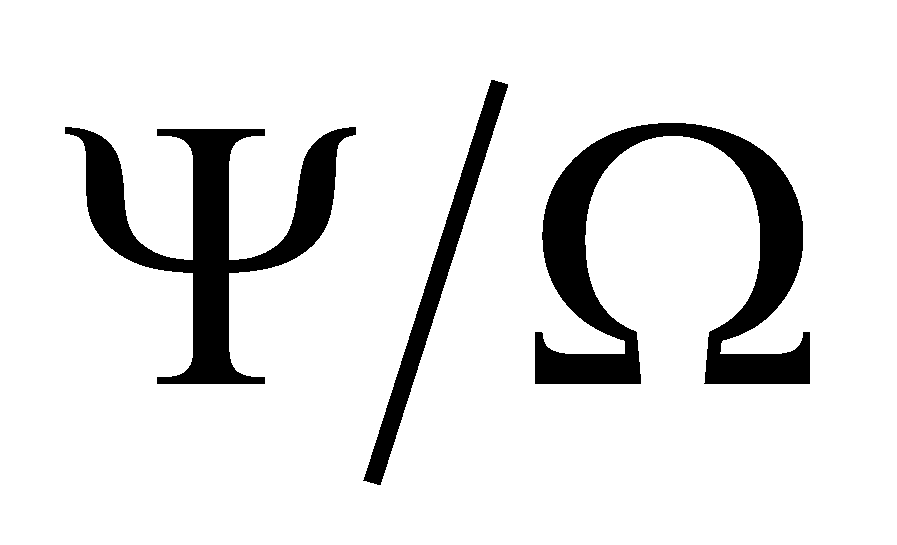is the average productivity of the volume unit and the limit of this ratio when all the points of the surfacetend to an internal point P, it is named the divergence of the vector field V in the point P.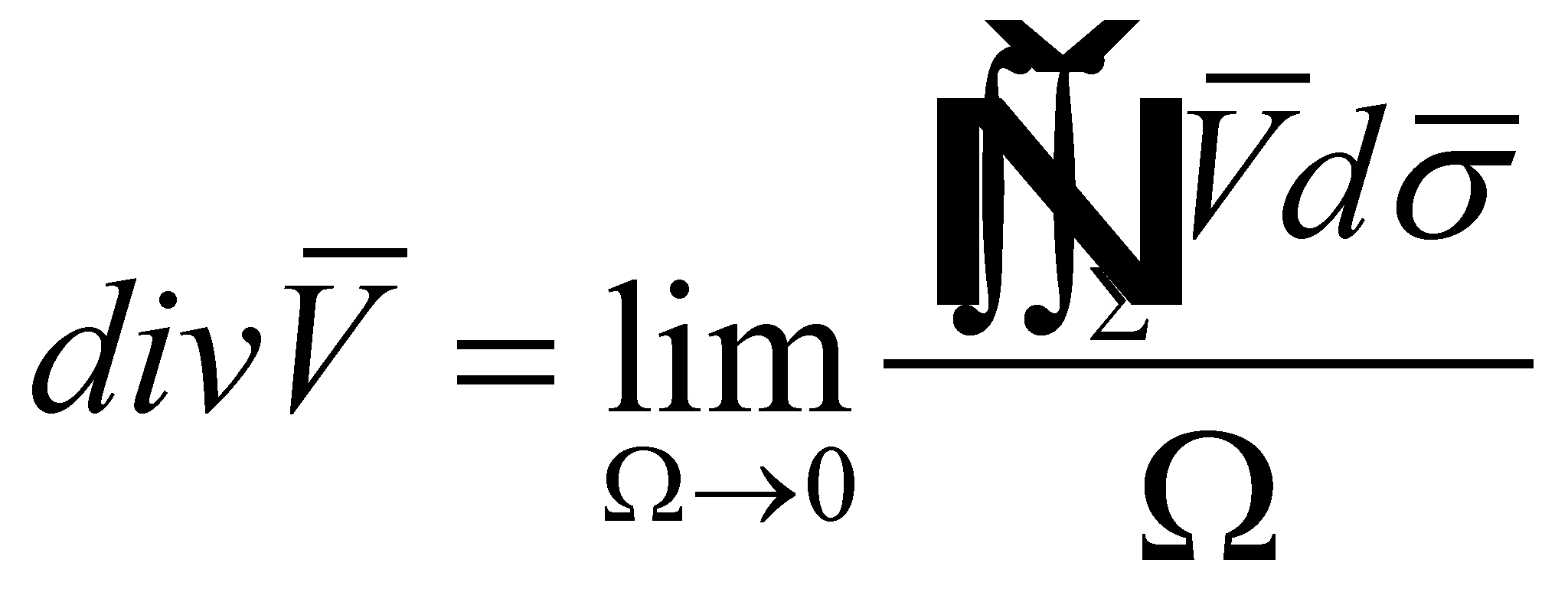(X.8.3)

Under the assumption that the partial derivatives ofare continuous in P, there is a limit which can be expressed by means of: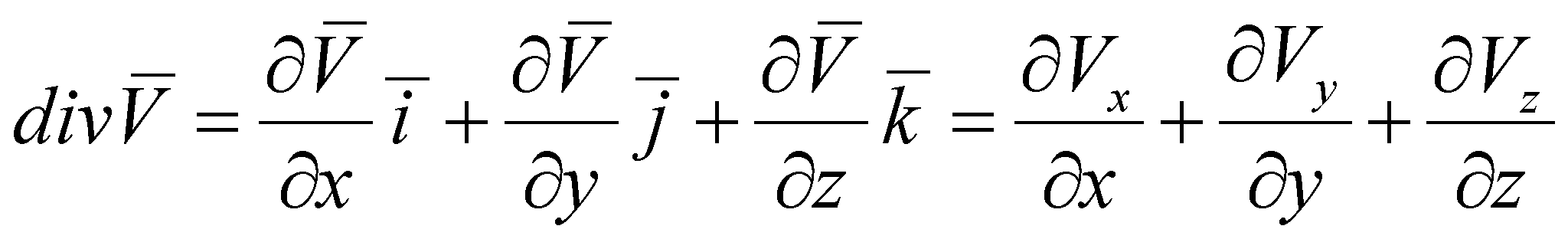(X.8.4)

1. The curl of a vector field,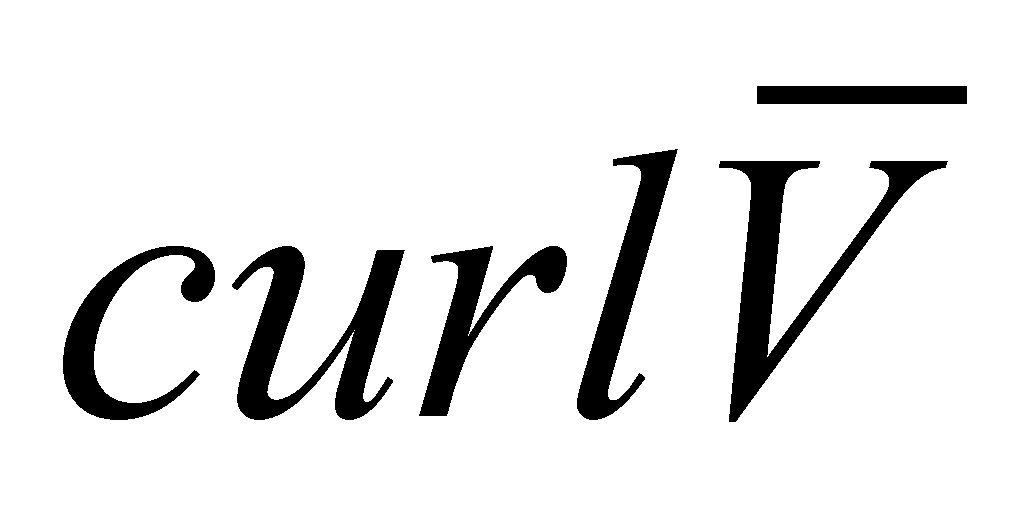, is defined by using the circulation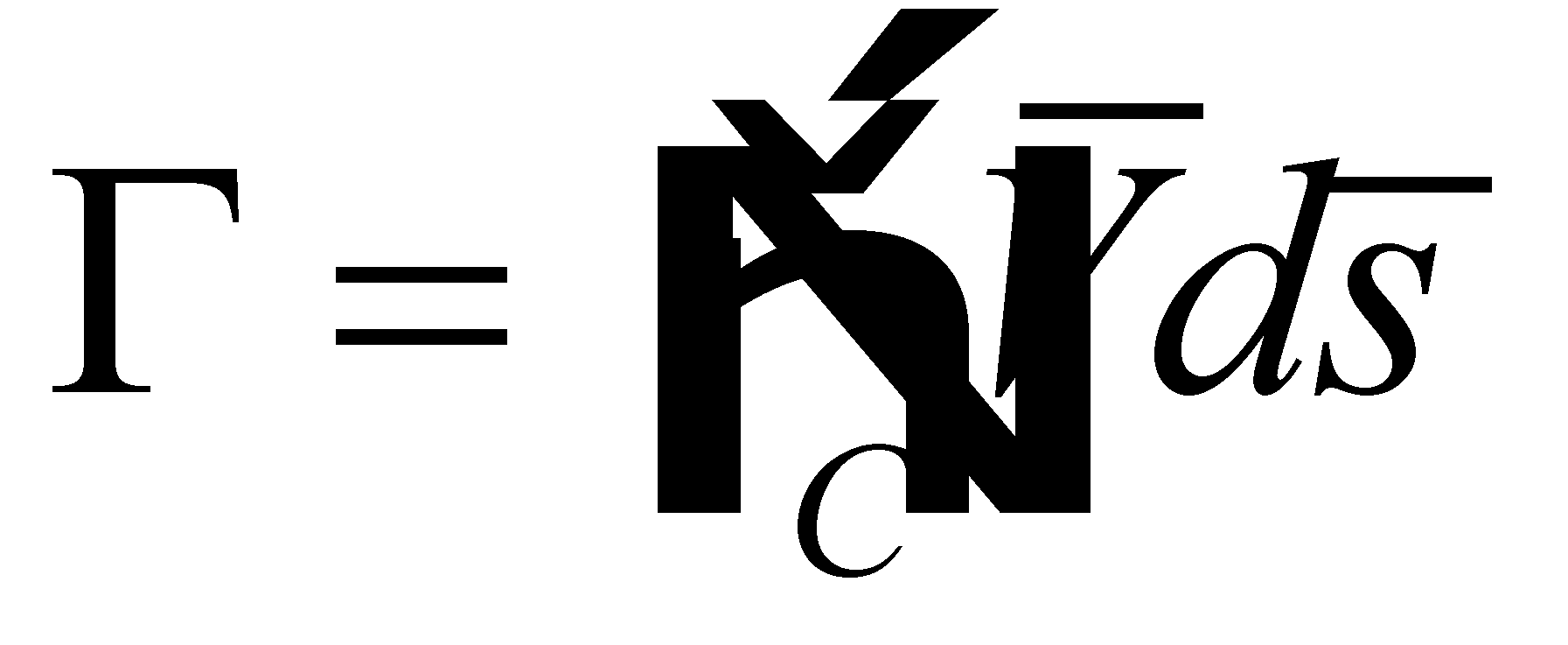on a confined curve C. A plane runs through the point P, having the versor of the normal line in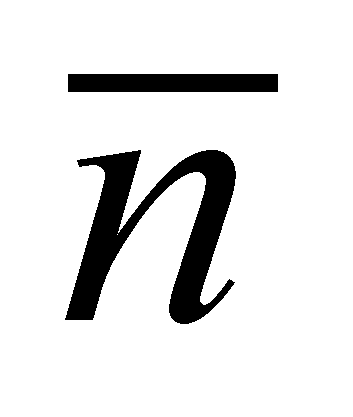. A confined curve C which surrounds the point P, placed in this plane delimit an area. It is known that the limit of the ratio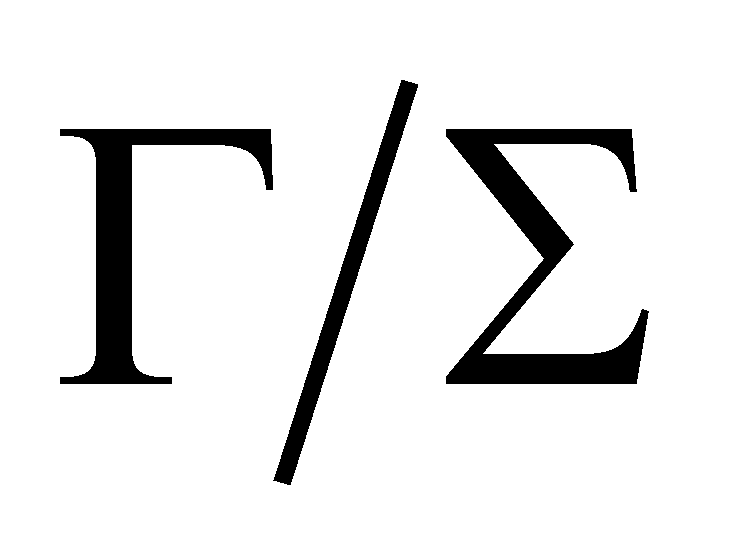when all the points of the curve C tend to P is the projection of a vector on the direction, that is a vector which is known as the curl of the fieldin the point P. Therefore,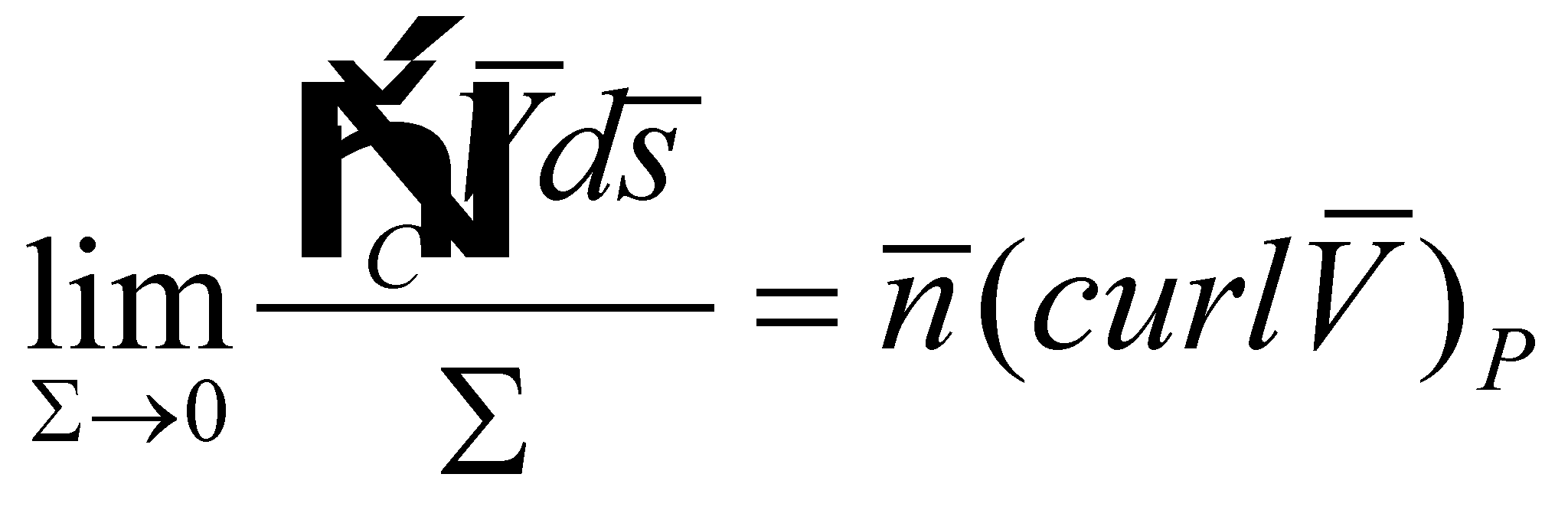(X.8.5)

where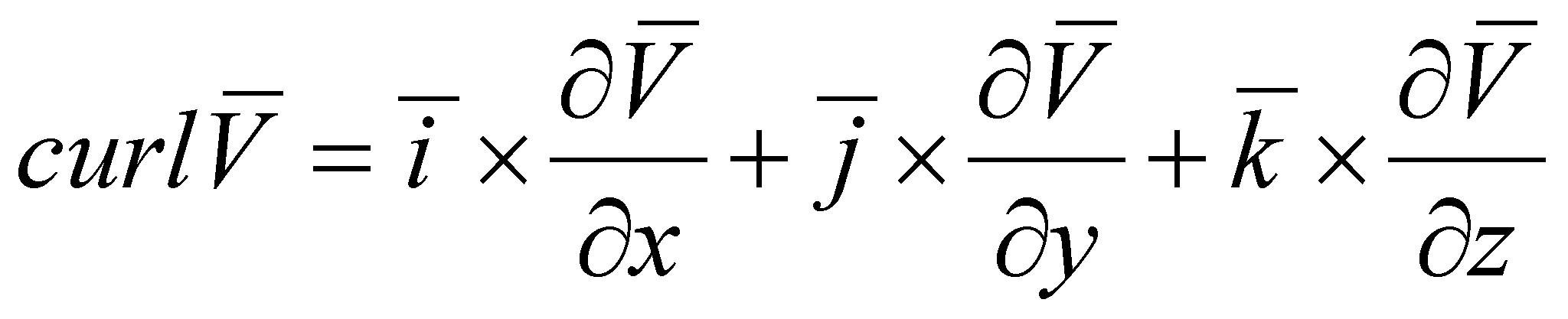(X.8.6)

Circulation on the curve C goes directly against(rule of the right screw). The curl may be also written as a symbolic determinant: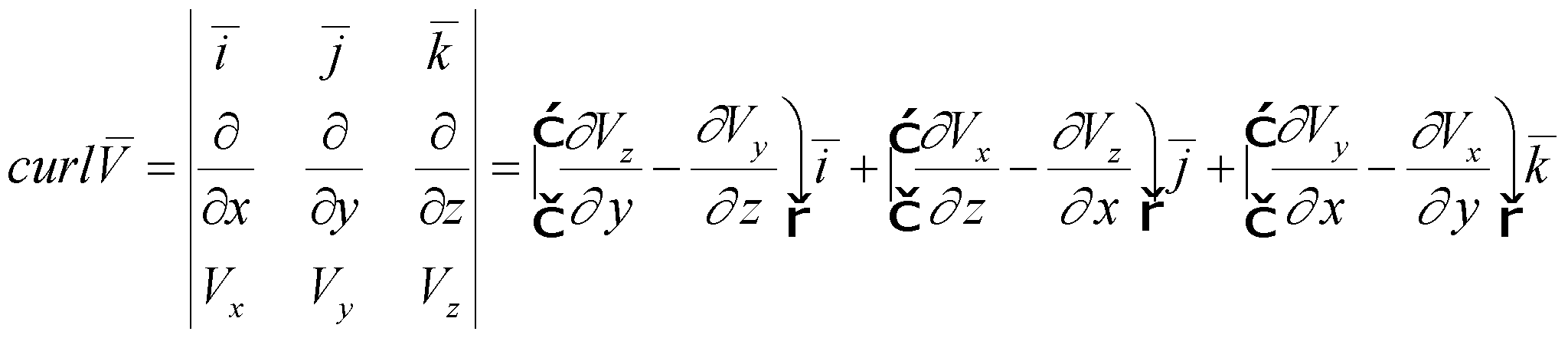(X.8.7)

1. The divergence’s integral formula (Gauss-Ostrogradski):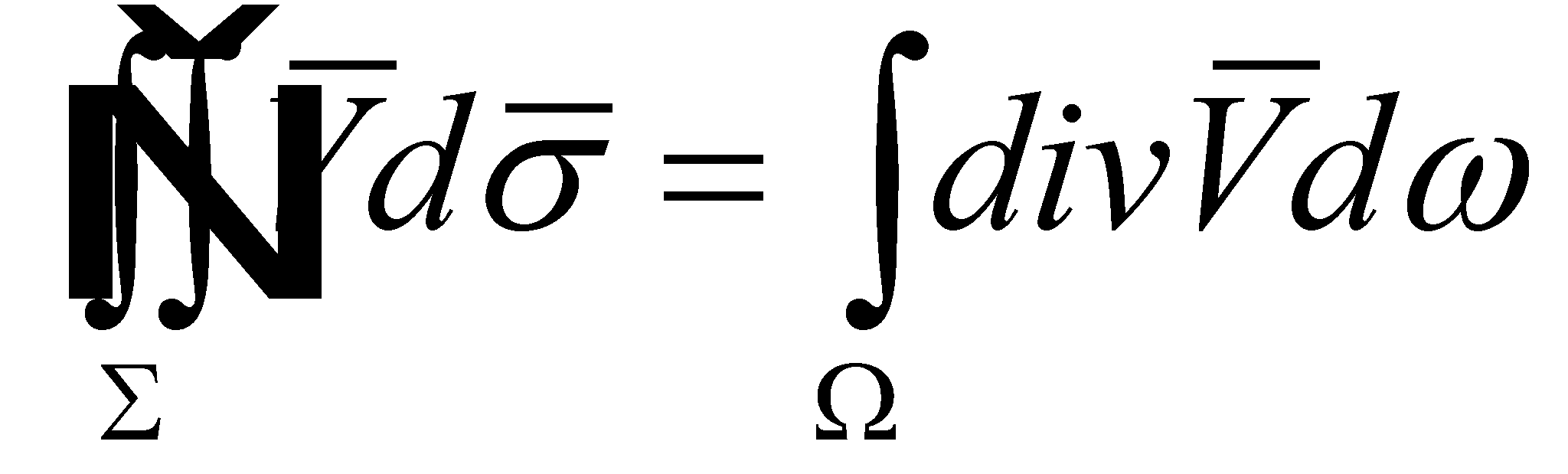(X.8.8)

whereis the confined area of the volume. The sense of the normal line on the oriented surface is considered to be positive to the outside. Other two relations are coming from the relation X.8.8, that is the curl’s integral formula: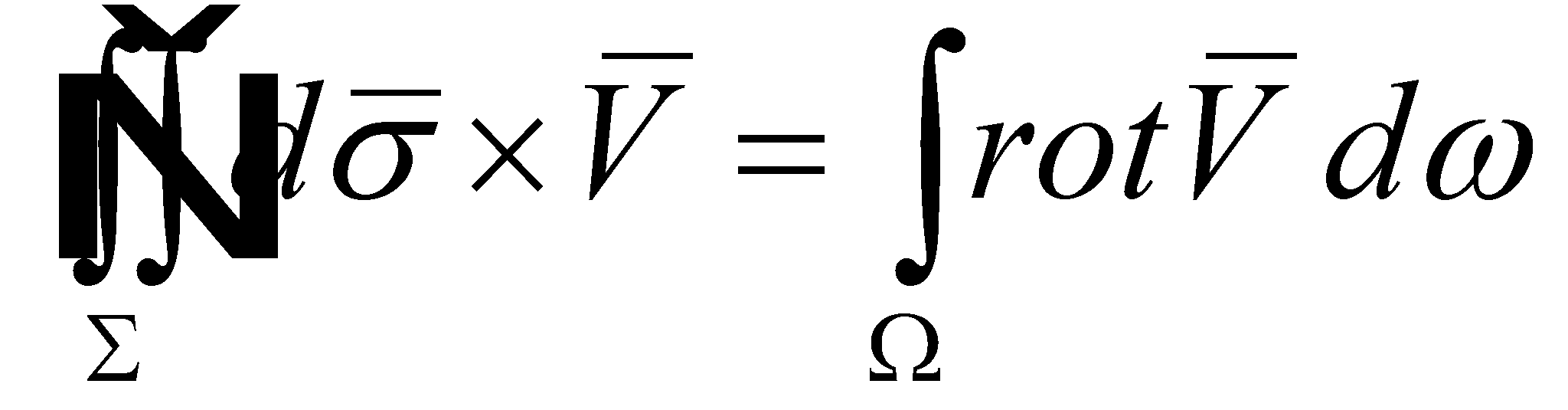(X.8.9)

which may be also written as: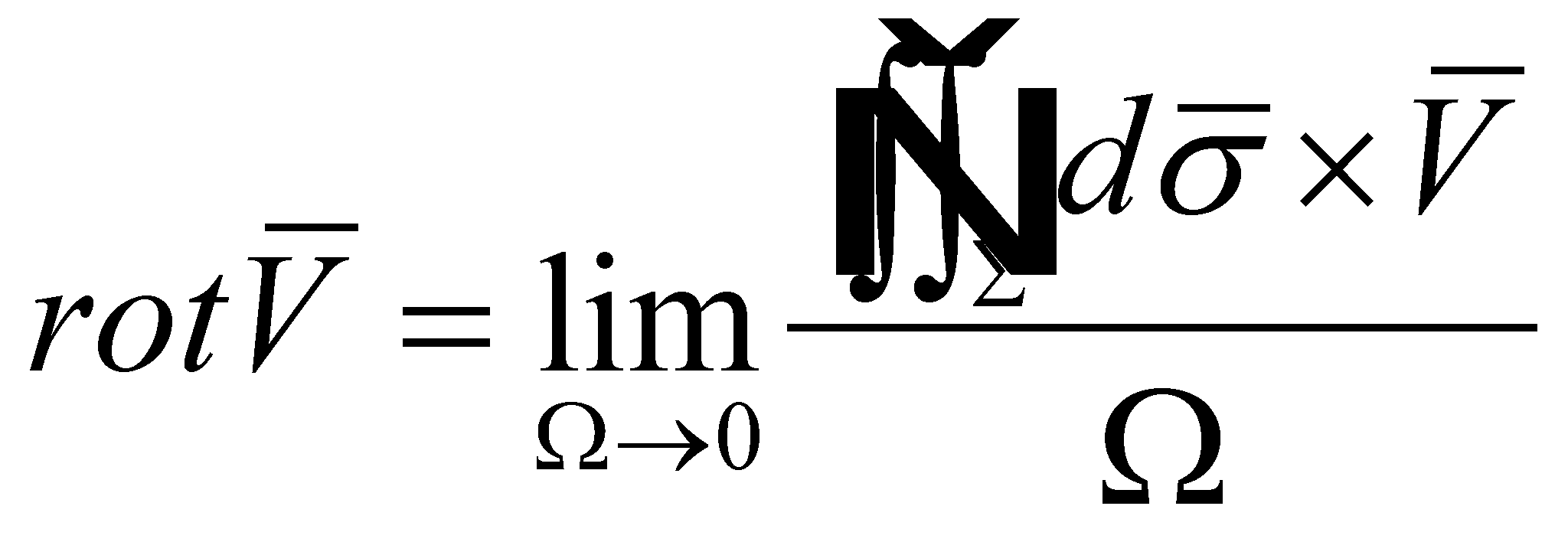(X.8.10)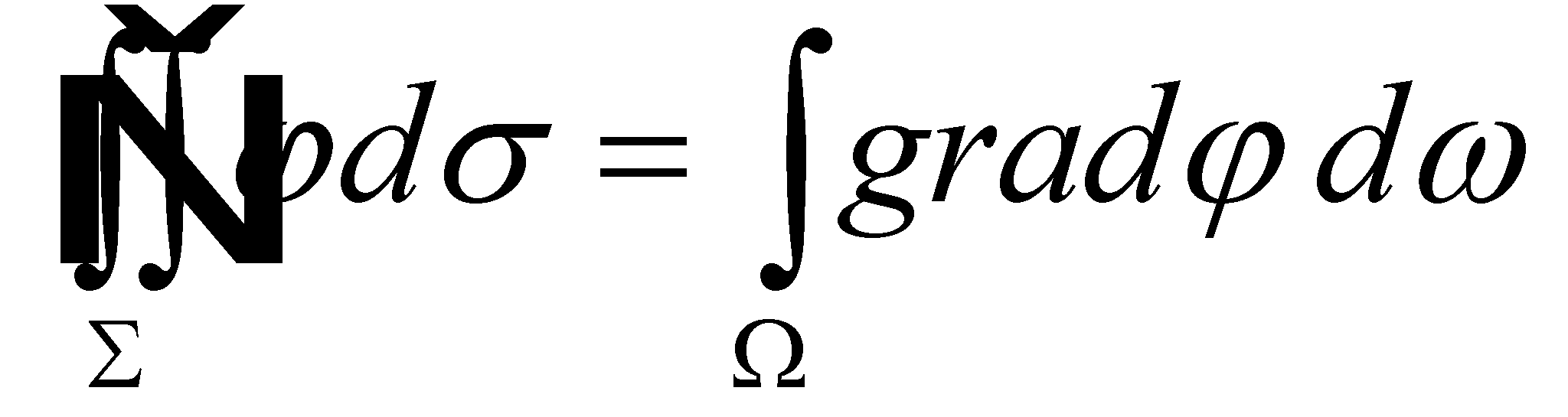(X.8.11)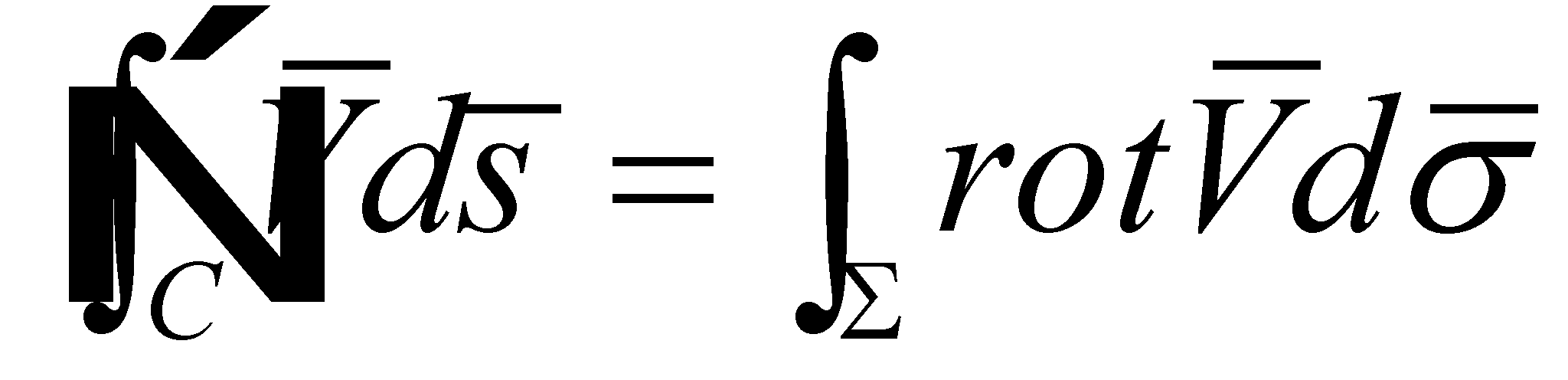(X.8.12)
whereis any surface limited by the confined curve C.